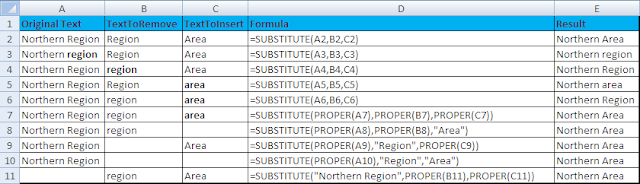### Excel Formula and Functions: SUBSTITUTE

ABOUT: SUBSTITUTE function substitutes(replaces) old_text with new_text. When you want to replace a certain text in a text string. SUBSTITUTE is different from REPLACE Function which replace any text (not specified string) which occurs at specific location. SUBSTITUTE finds the old_text and replace with new_text while REPLACE finds the position and number of characters to be replaced.

SYNTAX:
=SUBSTITUTE(OriginalText, TextToRemove, TextToInsert, instance_num)

OriginalText  → The Text or the reference to a cell containing text for which you want to substitute characters or text string.

TextToRemove → The text to be replaced with TextToInsert.

TextToInsert → The New Text - The Text you want instead of TextToRemove.

instance_num→ (It is Optional) - It specifies which occurence of TextToRemove you want to substitute with TextToInsert. If you specify instance_num, then only that instance of TextToRemove will be replaced. If it is omitted all instances of TextToRemove will be substituted with TextToInsert.

THINGS TO BE TAKEN CARE OF:-
1. This formula is case-sensitive. So be aware of the usage of UPPER, LOWER and PROPER Function also as your case may be.
2. Omitting instance_num will substitutes all the instances of the given Text or Cell Reference.

EXAMPLES:

Basic Use of Substitute FunctionView Advance use of Substitute Function on Microsoft Website:-

1 Formula to count the number of occurrences of a text string in a range
=SUM(LEN()-LEN(SUBSTITUTE(,"text","")))/LEN("text")

2 Formula to count the number of occurrences of a single character in one cell
=LEN()-LEN(SUBSTITUTE(,"a",""))

3 Formula to count the number of occurrences of a single character in a range
=SUM(LEN(range)-LEN(SUBSTITUTE(range,"a","")))

4 Formula to count the number of words separated by a character in a cell
=IF(LEN(TRIM())=0,0,LEN()- LEN(SUBSTITUTE(,,""))+1)

View the description of these examples of SUBSTITUTE function on Microsoft Website - http://support.microsoft.com/kb/187667